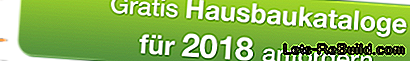# Calculation of roof tiles made easy

To cover a roof, you need a certain number of roof tiles. Nobody would like to sit on a rest or have already used up all the bricks after the half of the roof. Therefore, the number of bricks should be calculated exactly.

### Basis of the calculation is the roof area

Anyone who knows the lengths of his outer walls and the pitch of the roof can easily calculate the size of the roof area. Different lists show the factor with which you have to multiply the square meters of the house floor area in order to preserve the roof area.

#### example

A house 10 x 6 meters with a 35 degree pitched roof has a floor area of ​​60 square meters. You then have to multiply this by the factor 1.2207. This gives a roof area of ​​73,242 square meters.For comparison, if the roof had a 60-degree slope, the factor would be 2.000 and the roof area would be even 120.00 square meters.

### How many roof tiles does the roof need?

Most roof tiles are today manufactured in a standard size and have a cover size of 20 x 33 centimeters. Although there are even longer bricks, then you have to pay attention to the manufacturer's information regarding the cover size.

With this standardized measure, you need 15 roof tiles for one square meter. Correspondingly less are incurred when a different size is used.

Now you just have to multiply the number of square meters of the roof determined by this number. In our example, the 35-degree pitch roof would require 1099 tiles.

Tips & Tricks

Do not forget the additions if you break a frying pan or you have waste. About ten percent should be more than adequate in most cases as a blend.

Also do not forget dormers. Here a little more roof area can be created by the additional sides of the dormer.